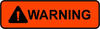### EIGEN-GRGS.RL02.MEAN-FIELD

EIGEN-GRGS.RL02.MEAN-FIELD is a static gravity field model, up to degree and order 160, based on 4.5 years of GRACE and LAGEOS data (March 2003 to September 2007). It includes time-variable terms up to degree and order 50.

• Reference epoch is 2005.00;
• Tide convention is « tide free »;
• The field is complete to degree and order 160;
• Up to degree and order 50, are present…
• drifts with the acronym « DOT »,
• annual sine and cosine terms with the acronym « S1A » and « C1A »,
• semi-annual sine and cosine terms with the acronym « S2A » and « C2A »,
• an offset, with the acronym « SUM », to take into account the permanent deformation of the gravity field associated with the December 2004 Sumatra earthquake. The principle of this correction is that if one works BEFORE December 24, 2004, one has to ADD this correction to the static gravity field; if one works AFTER this date, one has to IGNORE this correction. This means that EIGEN-GRGS.RL02.MEAN-FIELD is a « post-Sumatra » mean gravity field and that the Sumatra correction has only to be added if one works before the earthquake date of occurrence.

### EIGEN-GRGS.RL02.MEAN-FIELD.static_part.dg_050

We also provide the static-only part of EIGEN-GRGS.RL02.MEAN-FIELD, limited to maximum degree and order 50, under the name EIGEN-GRGS.RL02.MEAN-FIELD.static_part.dg_050. This is the same as the above-described model, but all time-dependant terms and all terms of spherical harmonic degree greater than 50 have been removed. To see the full temporal gravity variations, the 10-day solutions must be compared to this model (i.e. by subtracting this reference from the 10-day solutions).

### EIGEN-GRGS.RL02.MEAN-FIELD.static_part.dg_050 grids

In addition, we provide grids in geoid heights and equivalent water heights, with the following characteristics:

• Tides: “tide free”
• Degrees included: 02 to 50
• Parameters of the ellipsoid:
• a= 0.63781364600000E+07
• 1/f= 0.29825765000000E+03
• GM= 0.39860044150000E+15

### EIGEN-GRGS.RL02bis.MEAN-FIELD

EIGEN-GRGS.RL02bis.MEAN-FIELD is an extension of EIGEN-GRGS.RL02.MEAN-FIELD based on 8 years of GRACE and LAGEOS data. This is the reference gravity field for the GDR-D altimetric standards.

### EIGEN-6S2

EIGEN-6S2 is a result of the cooperation between GFZ (GeoForschungsZentrum, Potsdam) and CNES/GRGS on the « EIGEN » series of models. This model is proposed for the ITRF2013 standards.

Complete to degree and order 260, it is based on a GFZ internal GRACE+GOCE solution for the part between degree 51 and 260. The time-variable gravity (TVG) coefficients between degrees 2 and 50 are obtained from a regression on the GRGS-RL02 10-day time series (2003-2012). For degree 2 this TVG part is extended to 1985-2012 through the use of a GRGS SLR-only (Lageos+Lageos-2) solution between 1985 and 2003.

Outside of the measurements period (1985-2012 for degree 2, 2003-2012 for degrees 3 to 50), the gravity field is extrapolated with a zero-slope assumption.

The new modeling of the TVG part includes:

• Two annual and two semi-annual coefficients
• One bias and one drift for each year. (Note that the reference date of the bias is the beginning of the period of validity of the coefficient, i.e. the beginning of the year in most cases, see format). The bias and drift are in general coherent so that the result is a piece wise linear function, except in the case of earthquakes

The extended GRACE format is an extension of the official GRACE format for gravity fields. An explanation of the format is given here.

It is possible with our interactive tools to compute a static gravity field at any given date from this model.

In addition, you will find two software packages in Fortran90:

• a GRACE to ICGEM-V2 (and vice versa) format converter: here
• a kit to compute the gravity field at any given date from the new extended GRACE format: here
•On 2015/02/06 a new version of the two softwares has been uploaded. A bug had been uncovered in the subroutine « interpolate_gravity_model_at_date_t » in the file « read_write_interpolate.mod.f90 » of the kits. This bug will not affect any calculation done with the present mean gravity field models which have only one set of periodic terms per coefficient, but will affect the future mean gravity field models in which there will be one set of periodic terms per coefficient and per year. We strongly recommend to download the new versions of the softwares and install them in replacement of the old ones.